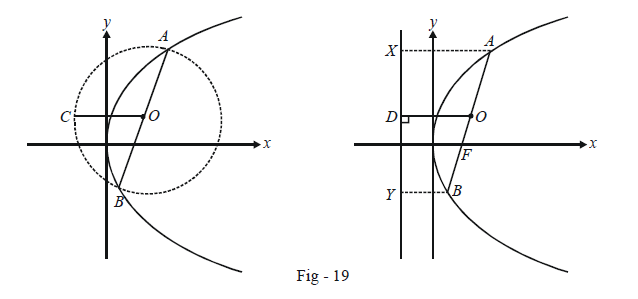# Length of Focal Chords

LENGTH OF ANY FOCAL CHORD: Through a point t, a focal chord is drawn in the parabola $${y^2} = 4ax$$ . The other end-point of this chord is, as described earlier, -\begin{align} \frac{1}{t}.\end{align}

Therefore, the length of this chord is

\begin{align}&{l_t} = \sqrt {{{\left( {a{t^2} - \frac{a}{{{t^2}}}} \right)}^2} + {{\left( {2at + \frac{{2a}}{t}} \right)}^2}} \\\\&= a\left( {t + \frac{1}{t}} \right)\sqrt {{{\left( {t - \frac{1}{t}} \right)}^2} + 4} \\\\&= a{\left( {t + \frac{1}{t}} \right)^2} \end{align}

The minimum length for any focal chord is evidently obtained when $$t = \pm 1,$$ which gives us the LR. Thus, the smallest focal chord in any parabola is its LR.

Example – 8

Prove that the circle described on any focal chord of a parabola as diameter will touch the directrix.

Solution: Let the equation of the parabola be $${y^2} = 4ax$$ so that the equation of the circle described on any focal chord as diameter can be written as

$(x - a{t^2})\left( {x - \frac{a}{{{t^2}}}} \right) + (y - 2at)\left( {y + \frac{{2a}}{t}} \right) = 0...\left( 1 \right)$

The directrix is x + a = 0. To show that it touches the circle given by (1), it is sufficient to show that substituting x = –a in (1) will give us only one value of y:

\begin{align}& \Rightarrow \quad ( - a - a{t^2})\left( { - a - \frac{a}{{{t^2}}}} \right) + (y - 2at)\left( {y + \frac{{2a}}{t}} \right) = 0\\\\&\Rightarrow \quad {a^2}\frac{{{{({t^2} + 1)}^2}}}{{{t^2}}} + {y^2} + \left( {\frac{{2a}}{t} - 2at} \right)y - 4{a^2} = 0\\\\&\Rightarrow \quad {y^2} + 2a\left( {\frac{1}{t} - t} \right)y + {a^2}{\left( {t - \frac{1}{t}} \right)^2} = 0\end{align}

This can evidently be written as a perfect square:

\begin{align}& \qquad \qquad {\left\{ {y - a\left( {t - \frac{1}{t}} \right)} \right\}^2} = 0\\ &\Rightarrow \qquad y = a\left( {t - \frac{1}{t}} \right)\end{align}

Only one value of y implies that the directrix touches the circle.

We can also prove the stated assertion geometrically simply by using the properties of a parabola. Consider any focal chord of the parabola, say AB; the next figures show the circle described on AB and the directrix of the parabola on different sketches.Let O be the centre of the circle so described. To show that it touches the directrix, we have to show its radius, say OC, is equal to the its perpendicular distance of O from the directrix.

Consider the second sketch. In trapezium XABY, since O is the mid point of AB, we have

$AX + BY = 2OD \qquad \dots(1)$

Since A lies on the parabola, we have by definition, AX = AF. Similarly, BY = BF. Thus,

$AX + BY = AF + BF = AB = 2AO \qquad \dots(2)$

(1) and (2) give OD = OA which implies OD is a radius of the circle, i.e. OD = OC. Thus, the circle touches the directrix.